# 2020 Full-time Interview CCJ’s Preparation (4): Machine Learning and Computer Vision Questions

see: 2020 Full-time Interview CCJ’s Preparation (1): Commonly Asked C++ Interview Questions, at this link.

see: 2020 Full-time Interview CCJ’s Preparation (2): Commonly Asked C++ Interview Questions in a Table, at this link.

see

## 1. Explain the difference between supervised and unsupervised machine learning?

In supervised machine learning algorithms, we have to provide labelled data, for example, prediction of stock market prices, whereas in unsupervised we need not have labelled data, for example, classification of emails into spam and non-spam.

## 2. Explain the difference between KNN and k-means clustering?

KNN is a supervised machine learning algorithm where we need to provide the labelled data to the model it then classifies the points based on the distance of the point from the nearest points.
Whereas, on the other hand, K-Means clustering is an unsupervised machine learning algorithm thus we need to provide the model with unlabelled data and this algorithm classifies points into clusters based on the mean of the distances between different points

## 3. What is the difference between classification and regression?

Classification is used to produce discrete results, classification is used to classify data into some specific categories .for example classifying e-mails into spam and non-spam categories.
Whereas, We use regression analysis when we are dealing with continuous data, for example predicting stock prices at a certain point of time.

## 4. How to ensure that your model is not overfitting?

• Keep the design of the model simple. Try to reduce the noise in the model by considering fewer variables and parameters.

• Cross-validation techniques such as K-folds cross validation help us keep overfitting under control.

• Regularization techniques such as LASSO (L1) and Ridge(L2) help in avoiding overfitting by penalizing certain parameters if they are likely to cause overfitting.

## 5. List the main advantage of Naive Bayes?

A Naive Bayes classifier converges very quickly as compared to other models like logistic regression. As a result, we need less training data in case of naive Bayes classifier.

## 6. Explain Ensemble learning.

In ensemble learning, many base models like classifiers and regressors are generated and combined together so that they give better results. It is used when we build component classifiers that are accurate and independent. There are sequential as well as parallel ensemble methods.

Ensemble learning helps improve machine learning results by combining several models. This approach allows the production of better predictive performance compared to a single model. Basic idea is to learn a set of classifiers (experts) and to allow them to vote.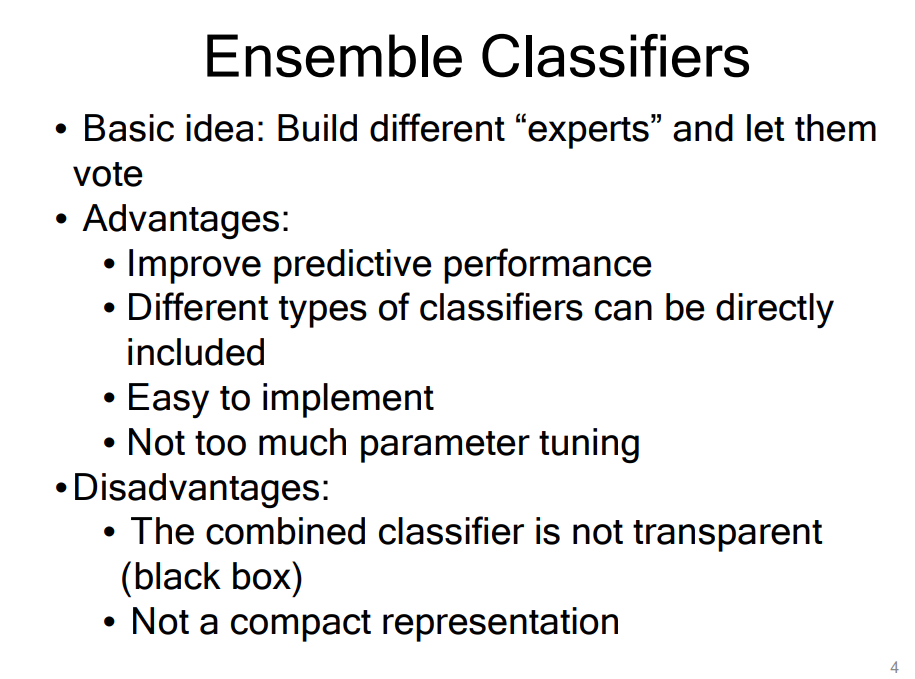• Why do they work?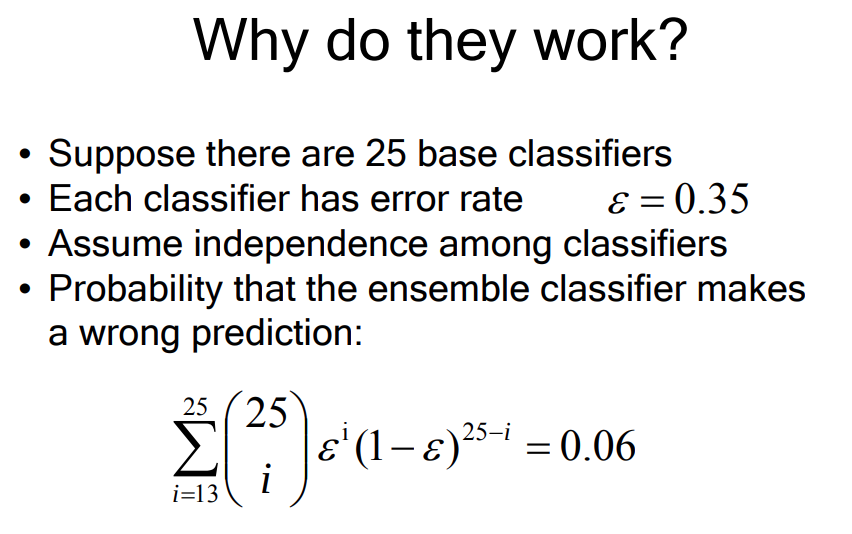## 7. Types of Ensemble Methods:

Ensemble Methods: Predict class label for unseen data by aggregating a set of predictions (classifiers learned from the training data)

Types of Ensemble Methods: bagging, random forests and boosting.

### 1) Bagging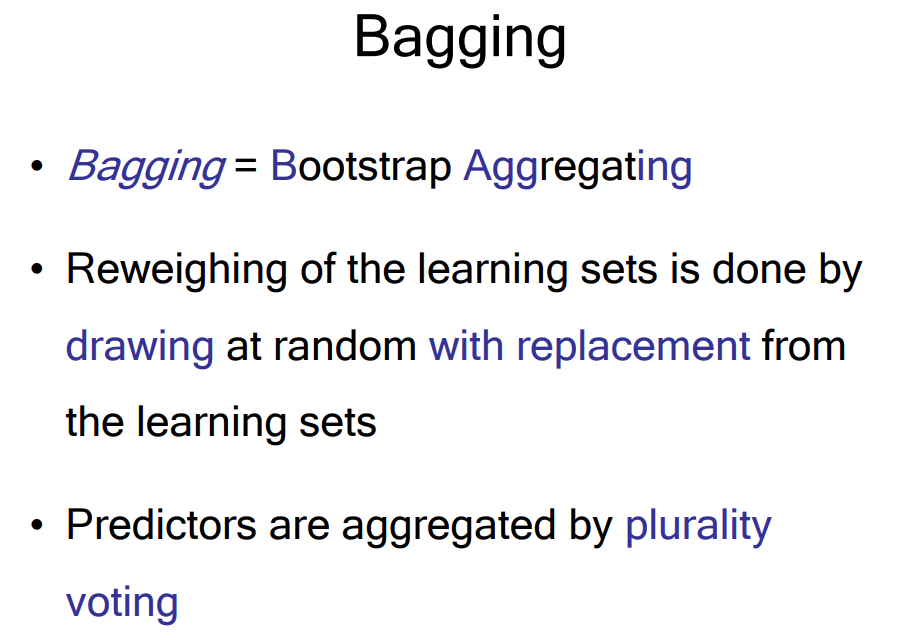• Bagging works because it reduces variance by voting/averaging

• In some pathological hypothetical situations the overall error might increase

• Usually, the more classifiers the better

• Problem: we only have one dataset

• Solution: generate new ones of size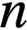by bootstrapping, i.e. sampling with replacement

• Can help a lot if data is noisy;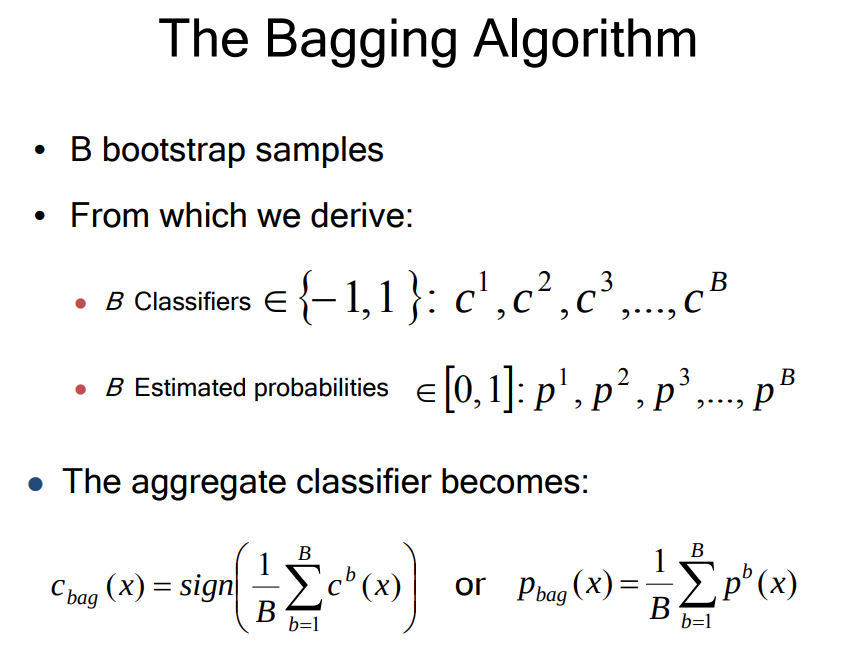• Bagging is performed in parallel.

### 2) Random Forests

• Random Forest is an extension over bagging.

• Each classifier in the ensemble is a decision tree classifier and is generated using a random selection of attributes at each node to determine the split.

• During classification, each tree votes and the most popular class is returned.

• A forest is an ensemble of trees. The trees are all slightly different from one another.

• Important Parameters:

• input data point: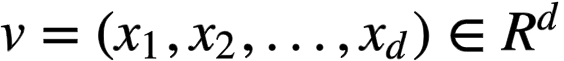• Node objective function (train): The “energy” to be minimized when training the j-th split node, e.g., information gain

• Stopping criteria (training), e.g., max tree depth = D, When to stop growing a tree during training;

• Tree depth D: (a) Big D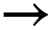overfitting (i.e., too much model capacity, model overfits the training data) and (b) Small Dunderfitting (i.e., too little model capacity);

• Forest size: T, Total number of trees in the forest;

How to achieve randomness?

• 1) Randomness Model: Bagging (randomizing the training set)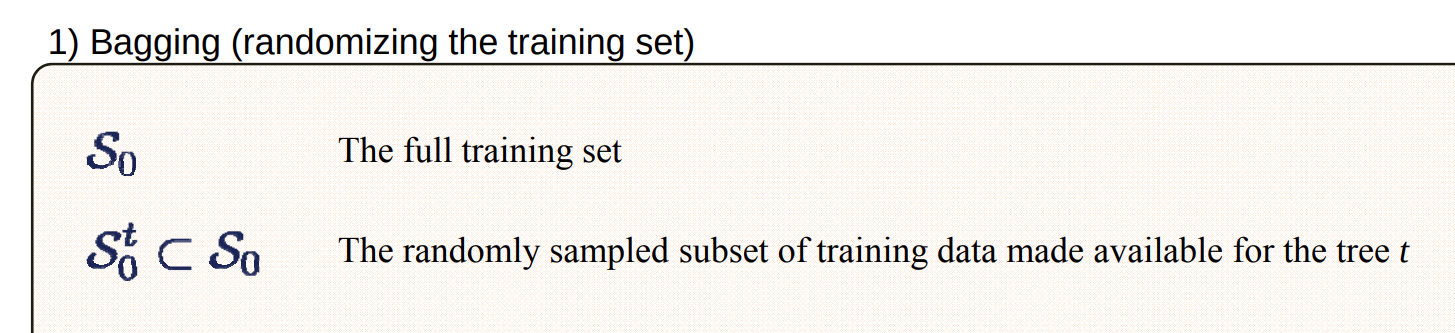• 2) Randomness Model: Randomized node optimization (RNO)